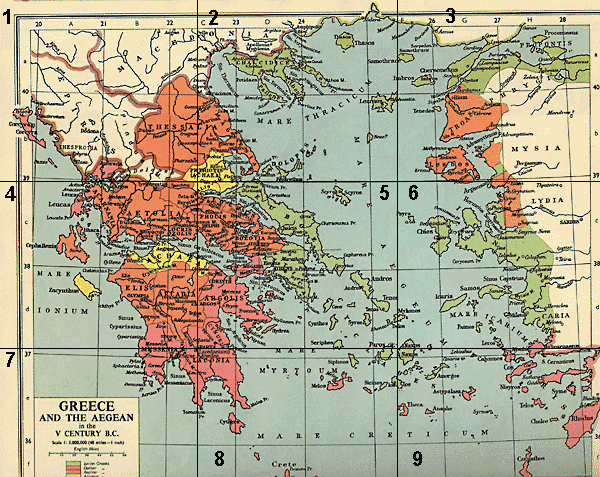Click on an area of the map below to see a closeup (some squares overlap)

 Square 1 Square 2 Square 3 Square 4 Square 5 Square 6 Square 7 Square 8 Square 9
 Amphipolis (sq.2) Potidaea (sq. 2) Athens (sq. 5) Lesbos (sq. 3) Sparta (sq. 5) Corinth (sq. 5) Sparta (sq. 7) Delphi (sq. 5) Argos (sq. 5) Olympia (sq. 4) Smyrna (sq. 6) Mount Olympus (sq. 2) Ilium (sq. 3) Ithica (sq. 4) Mycenae (sq. 5)